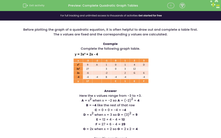### Comprehensive & curriculum aligned

In this worksheet, students will learn how to complete tables for quadratic graphs.Key stage:  KS 3

Curriculum topic:   Algebra

Curriculum subtopic:   Use Linear Equations (Two Variables) and Gradients

Difficulty level:#### Worksheet Overview

Before plotting the graph of a quadratic equation, it is often helpful to draw out and complete a table first.

The x values are fixed and the corresponding y values are calculated.

Example

Complete the following graph table.Here the x values range from -3 to +3.

A = x2 when x = -2 so A = (-2)2 = 4

B = -4 like the rest of that row

C = 0 + 0 + -4 = -4

D = x2 when x = 3 so D = (3)2 = 9

E = 12 + 4 - 4 = 12

F = 27 + 6 - 4 = 29

G = 2x when x = 2 so G = 2 x 2 = 4

Now it's over to you!

### What is EdPlace?

We're your National Curriculum aligned online education content provider helping each child succeed in English, maths and science from year 1 to GCSE. With an EdPlace account you’ll be able to track and measure progress, helping each child achieve their best. We build confidence and attainment by personalising each child’s learning at a level that suits them.

Get started